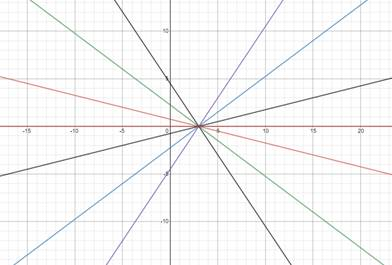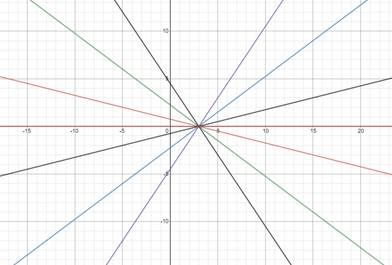# Graph the equation in same viewing rectangle. Find common trait.### Precalculus: Mathematics for Calcu...

6th Edition
Stewart + 5 others
Publisher: Cengage Learning
ISBN: 9780840068071### Precalculus: Mathematics for Calcu...

6th Edition
Stewart + 5 others
Publisher: Cengage Learning
ISBN: 9780840068071

#### Solutions

Chapter 1.10, Problem 43E
To determine

## Graph the equation in same viewing rectangle. Find common trait.

Expert SolutionAll the lines of given equations pass through same point, i.e., (3,0) .

### Explanation of Solution

Given information:

y=m(x3)

m=0,±0.25,±0.75,±1.5All the line of these equations pass through same point, (3,0)

### Have a homework question?

Subscribe to bartleby learn! Ask subject matter experts 30 homework questions each month. Plus, you’ll have access to millions of step-by-step textbook answers!